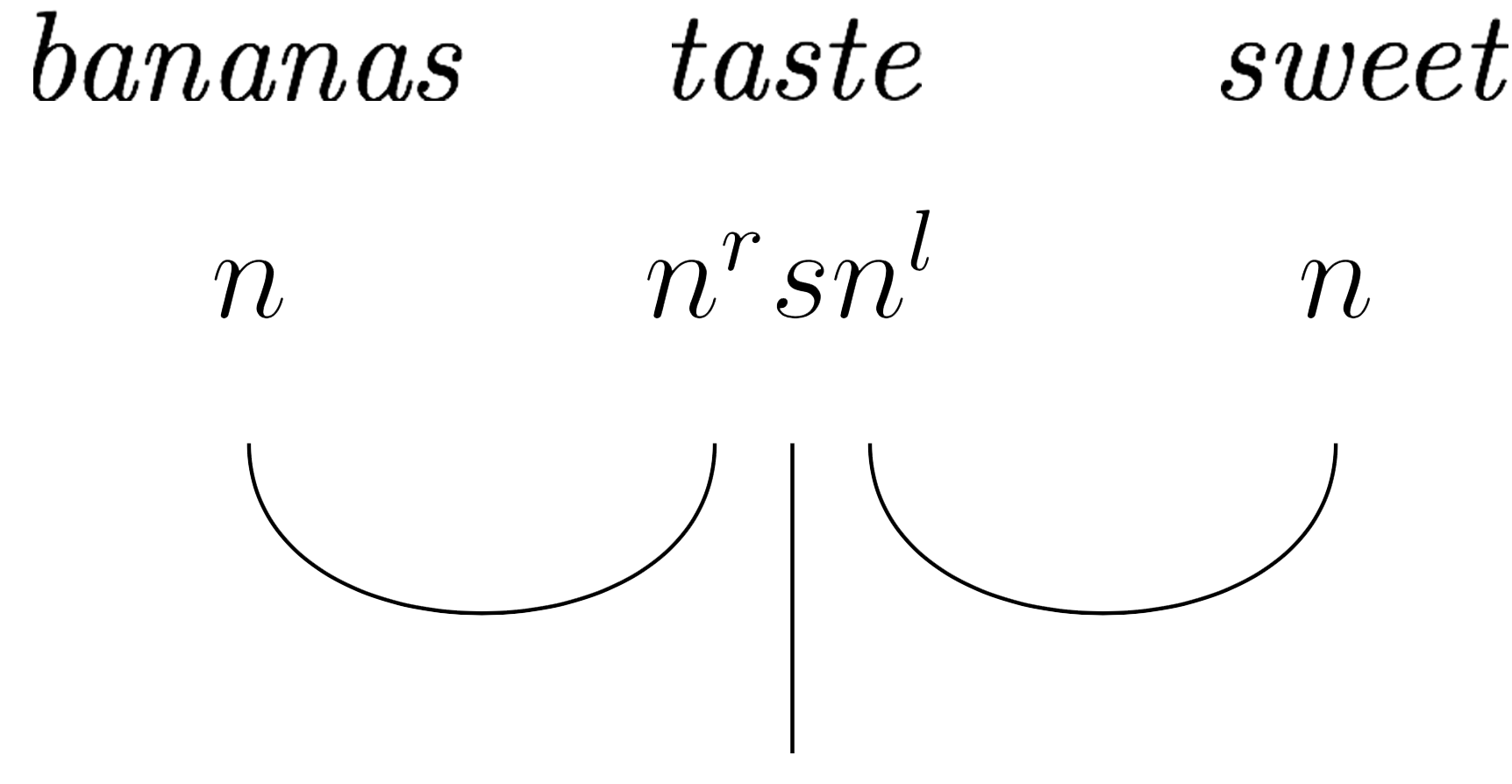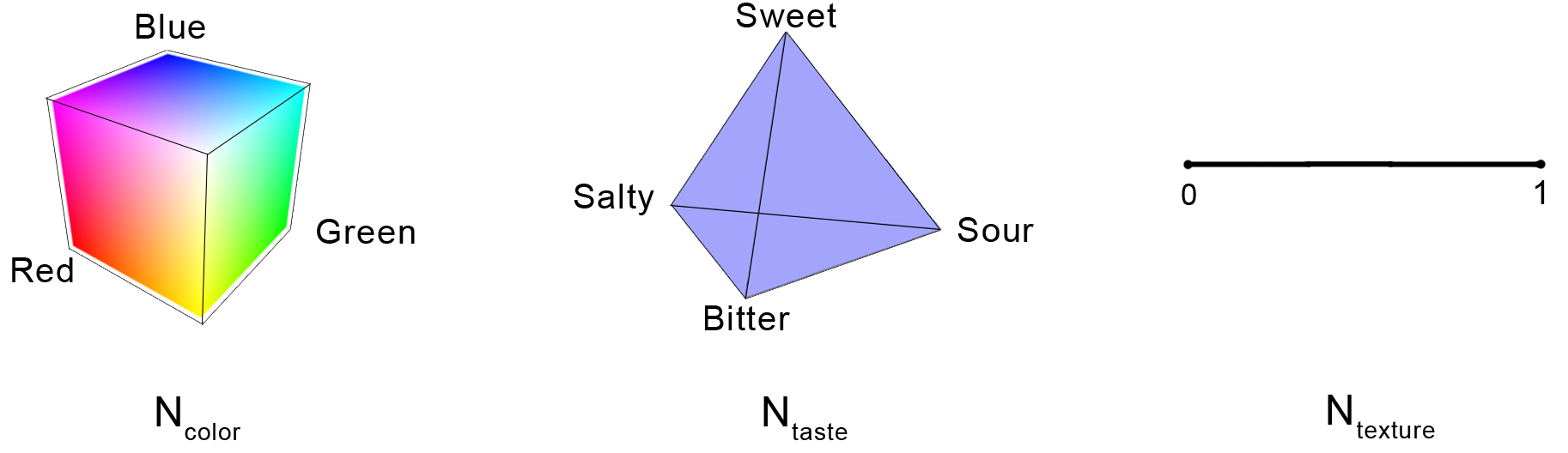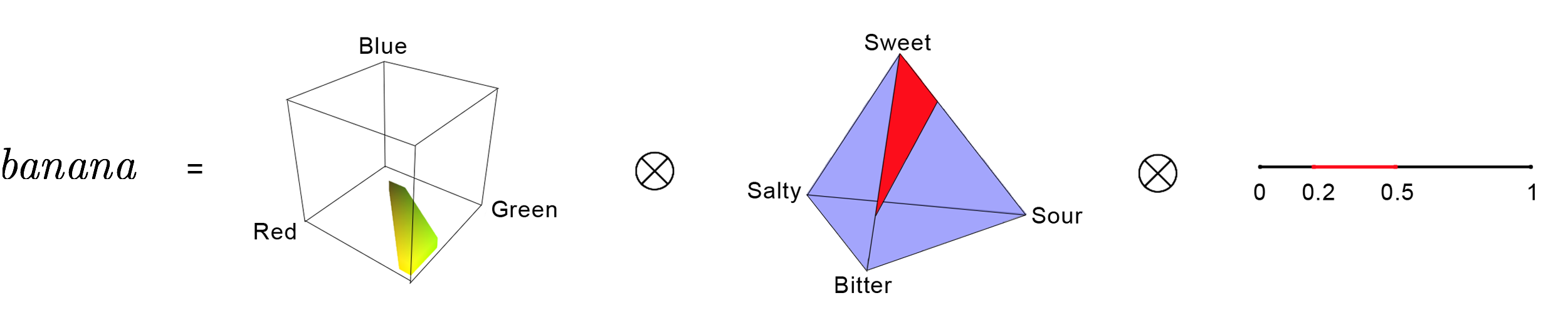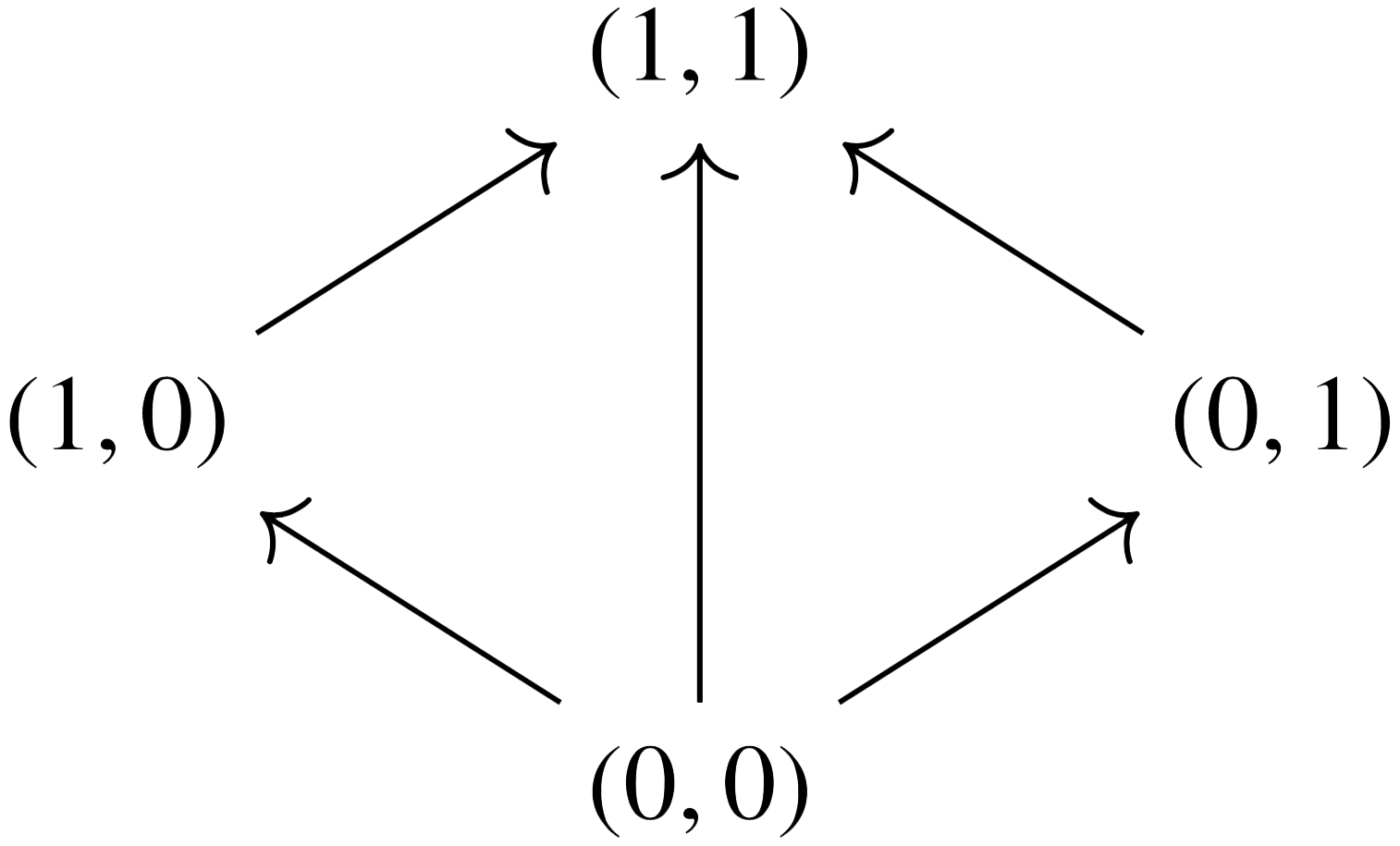## March 10, 2018

### Cognition, Convexity, and Category Theory

#### Posted by John BaezRecently in the Applied Category Theory Seminar our discussions have returned to modeling natural language, this time via Interacting Conceptual Spaces I by Joe Bolt, Bob Coecke, Fabrizio Genovese, Martha Lewis, Dan Marsden, and Robin Piedeleu. In this paper, convex algebras lie at the heart of a compositional model of cognition based on Peter Gärdenfors’ theory of conceptual spaces. We summarize the ideas in today’s post.

Sincere thanks go to Brendan Fong, Nina Otter, Fabrizio Genovese, Joseph Hirsh, and other participants of the seminar for helpful discussions and feedback.

## Introduction

A few weeks ago here at the Café, Cory and Jade summarized the main ideas behind the DisCoCat model, i.e. the categorical compositional distributional model of meaning developed in a 2010 paper by Coecke, Sadrzadeh, and Clark. Within the comments section of that blog entry, Coecke noted that the DisCoCat model is essentially a grammatical quantum field theory — a functor (morally) from a pregroup to finite dimensional real vector spaces. In this model, the meaning of a sentence is determined by the meanings of its constituent parts, which are themselves represented as vectors with meanings determined statistically. But as he also noted,

Vector spaces are extremely bad at representing meanings in a fundamental way, for example, lexical entailment, like tiger < big cat < mammal < animal can’t be represented in a vector space. At Oxford we are now mainly playing around with alternative models of meaning drawn from cognitive science, psychology and neuroscience. Our Interacting Conceptual Spaces I is an example of this….

This (ICS I) is the paper that we discuss in today’s blog post. It presents a new model in which words are no longer represented as vectors. Instead, they are regions within a conceptual space, a term coined by cognitive scientist Peter Gärdenfors in Conceptual Spaces: The Geometry of Thought. A conceptual space is a combination of geometric domains where convexity plays a key role. Intuitively, if we have a space representing the concept of fruit, and if two points in this space represent banana, then one expects that every point “in between” should also represent banana. The goal of ICS I is to put Gärdenfors’ idea on a more formal categorical footing, all the while adhering to the main principles of the DisCoCat model. That is, we still consider a functor out of a grammar category, namely the pregroup $\mathsf{Preg}(n,s)$, freely generated by noun type $n$ and sentence type $s$. (But in light of Preller’s argument as mentioned previously, we use the word functor with caution.) The semantics category, however, is no longer vector spaces but rather $\mathsf{ConvexRel},$ the category of convex algebras and convex relations. We make these ideas and definitions precise below.

## Preliminaries

A convex algebra is, loosely speaking, a set equipped with a way of taking formal finite convex combinations of its elements. More formally, let $A$ be a set and let $D(A)$ denote the set of formal finite sums $\sum_i p_i a_i$ of elements of $A,$ where $p_i\in\mathbb{R}_{\geq 0}$ and $\sum_i p_i=1.$ (We emphasize that this sum is formal. In particular, $A$ need not be equipped with a notion of addition or scaling.) A convex algebra is a set $A$ together with a function $\alpha\colon D(A)\to A$, called a “mixing operation,” that is well-behaved in the following sense:

• the convex combination of a single element is itself, and
• the two ways of evaluating a convex combination of a convex combination are equal.

For example, every convex subspace of $\mathbb{R}^n$ is naturally a convex algebra. (And we can’t resist mentioning that convex subspaces of $\mathbb{R}^n$ are also examples of algebras over the operad of topological simplices. But as we learned through a footnote in Tobias Fritz’s Convex Spaces I, it’s best to stick with monads rather than operads. Indeed, a convex algebra is an Eilenberg-Moore algebra of the finite distribution monad.) Join semilattices also provide an example of convex algebras. A finite convex combination of elements $a_i$ in the lattice is defined to be the join of those elements having non-zero coefficients: $\sum_i p_i a_i:=\vee_i \{a_i:p_i\neq 0\}$. (In particular, the coefficients play no role on the right-hand side.)

Given two convex algebras $(A,\alpha)$ and $(B,\beta)$, a convex relation is a binary relation $R\subseteq A\times B$ that respects convexity. That is, if $R(a_i,b_i)$ for all $i=1,\ldots,n$ then $R\left(\alpha(\sum_{i=1}^n p_i a_i),\beta(\sum_{i=1}^n p_i b_i)\right)$. We then define $\mathsf{ConvexRel}$ to be the category with convex algebras as objects and convex relations as morphisms. Composition and identities are as for usual binary relations.

Now since in this model, the category of vector spaces is being replaced by $\mathsf{ConvexRel}$, one hopes that (in keeping with the spirit of the DisCoCat model), the latter admits a symmetric monoidal compact closed structure. Indeed it does.

• $\mathsf{ConvexRel}$ has a symmetric monoidal structure given by the Cartesian product: We use $(A,\alpha)\otimes(B,\beta)$ to denote the set $A\times B$ equipped with mixing operation given by \begin{aligned} D(A\times B)&\longrightarrow A\times B\\ \sum p_i(a_i,b_i)&\mapsto \left(\alpha(\sum p_i a_i),\beta(\sum p_i b_i)\right). \end{aligned} The monoidal unit is the one-point set $\star$ which has a unique convex algebra structure. We’ll denote this convex algebra by $I.$
• Each object in $\mathsf{ConvexRel}$ is self-dual, and cups and caps are given as follows: \begin{aligned} \eta_A\colon I \to(A,\alpha)\otimes(A,\alpha) \quad &\text{ is the relation} \quad \{(\star,(a,a)):a\in A)\}\\ \epsilon_A\colon (A,\alpha)\otimes(A,\alpha)\to I \quad &\text{ is the relation} \quad \{((a,a),\star):a\in A\} \end{aligned}

The compact closed structure guarantees that $\mathsf{ConvexRel}$ fits nicely into the DisCoCat framework: words in a sentence are assigned types according to a chosen pregroup grammar, and a sentence is deemed grammatical if it reduces to type $s$. Moreover, these type reductions in $\mathsf{Preg}(n,s)$ give rise to corresponding morphisms in $\mathsf{ConvexRel}$ where the meaning of the sentence can be determined. We’ll illustrate this below by computing the meaning of the sentence

$b a n a n a s\; t a s t e\; s w e e t.$

To start, note that this sentence is comprised of three grammar types:and each corresponds to a different conceptual space $\text{noun, }\; n \rightsquigarrow N \qquad\qquad \text{sentence, }\; s \rightsquigarrow S \qquad\qquad \text{verb, }\; n^r s n^l \rightsquigarrow N\otimes S \otimes N$ which we describe next.

## Computing Meaning

### The Noun Space $N$

A noun is a state $I\to N$, i.e. a convex subset of the noun space $N$. Restricting our attention to food nouns, the space $N$ is a product of color, taste, and texture domains: $N=N_{\text{color}}\otimes N_{\text{taste}}\otimes N_{\text{texture}} \subset \mathbb{R}^8$ where

• $N_{\text{color}}$ is the RGB color cube, i.e. the set of all triples $(R,G,B)\in [0,1]^3$.
• $N_{\text{taste}}$ is the taste tetrahedron, i.e. the convex hull of four basic tastes: sweet, sour, bitter, and salty.
• $N_{\text{texture}}$ is the unit interval $[0,1]$ where 0 represents liquid and 1 represents solid.The noun banana is then a product of three convex subregions of $N$:That is, banana is the product of a yellow/green region, the convex hull of three points in the taste tetrahedron, and a subinterval of the texture interval. Other foods and beverages, avocados, chocolate, beer, etc. can be expressed similarly.

### The Sentence Space $S$

The meaning of a sentence is a convex subset of a sentence space $S$. Here, $S$ is chosen as a simple-yet-sensible space to capture one’s experience when eating and drinking. It is the join semilattice on four pointswhere in the first component, 0 = negative and 1 = positive, while in the second component, 0 = not surprising and 1 = surprising. For instance, $(0,1)$ represents negative and surprising while the convex subset $\{(1,1),(1,0)\}$ represents positive.

### The Verb Space $N\otimes S\otimes N$

A transitive verb is a convex subset of $N\otimes S\otimes N$. For instance, if we suppose momentarily that we live in a world in which one can survive on bananas and beer alone, then the verb taste can be represented by \begin{aligned} t a s t e &= \text{Conv}( \{g r e e n\; b a n a n a \otimes\{(0,0)\}\otimes b i t t e r\}\\ &\,\cup \{g r e e n\; b a n a n a\otimes\{(1,1)\}\otimes s w e e t\}\\ &\,\cup \{y e l l o w\; b a n a n a\otimes\{(1,0)\}\otimes s w e e t\}\\ &\,\cup \{b e e r \otimes\{(0,1)\}\otimes s w e e t\}\\ &\,\cup \{b e e r\otimes\{(1,0)\}\otimes b i t t e r\}) \end{aligned} where Conv denotes the convex hull of the argument. Here, green is an intersective adjective, so green banana is computed by taking the intersection of the banana space with the green region of the color cube. Likewise for yellow banana.

## Tying it all together

Finally, we compute the meaning of bananas taste sweet, which has grammar type reduction $n(n^r s n^l)n\leq (nn^r )s\leq s.$ In $\mathsf{ConvexRel}$, this corresponds to the following morphism: $\overset{\text{bananas}}{N}\otimes\;\; \overset{\text{taste}}{N\;\; \otimes \;\; S\;\; \otimes \;\;N}\;\; \otimes \overset{\text{sweet}}{N}\;\;\;\overset{\epsilon_N\otimes 1_S\otimes\epsilon_N}{\longrightarrow}\;\;\; S$ \begin{aligned} b a n a n a s\; t a s t e\; s w e e t &= (\epsilon_N\otimes 1_S\otimes \epsilon_N)(b a n a n a s \otimes t a s t e \otimes s w e e t)\\ &=(\epsilon_N\otimes 1_S)(b a n a n a\otimes (g r e e n\; b a n a n a \otimes \{(1,1)\} \\ &\qquad\qquad\qquad\qquad\quad \cup y e l l o w\; b a n a n a \otimes\{(1,0)\} \\ &\qquad\qquad\qquad\qquad\quad \cup b e e r\otimes\{(0,1)\}))\\ &=\{(1,1),(1,0)\}\\ &= p o s i t i v e \end{aligned} Note that the rightmost $\epsilon_N$ selects subsets of the taste space that include sweet things and then deletes “sweet.” The leftmost $\epsilon_N$ selects subsets of the taste space that include banana and then deletes “banana.” We are left with a convex subset of $S$, i.e. the meaning of the sentence.

## Closing Remarks

Although not shown in the example above, one can also account for relative pronouns using certain morphisms called multi-wires or spiders (these arise from commutative special dagger Frobenius structures). The authors also give a toy example from the non-food world by modeling movement of a person from one location to another, using time and space to define new noun, sentence, and verb spaces.

In short, the conceptual spaces framework seeks to capture meaning in a way that resembles human thought more closely than the vector space model. This leaves us to puzzle over a couple of questions: 1) Do all concepts exhibit a convex structure? and 2) How might the conceptual spaces framework be implemented experimentally?

Posted at March 10, 2018 6:51 PM UTC

TrackBack URL for this Entry:   https://golem.ph.utexas.edu/cgi-bin/MT-3.0/dxy-tb.fcgi/3026

### Re: Cognition, Convexity, and Category Theory

Nice!

So why is it best to stick with the monad rather than work with Tom Leinster’s operad in this situation? I’m too lazy to find the footnote in Tobias Fritz’s paper.

I like Tom’s operad $\mathbf{P}$, whose operations are convex linear combinations:

$\mathbf{P}_n = \{ p \in \mathbb{R}^n : \; p_i \ge 0, \; \sum_{i = 1}^n p_i = 1 \}$

I think that the surprising theorem relating Tom’s operad and Shannon information (also called Shannon entropy), together with material you’re discussing on convex sets and ‘meaning’, might reveal an interesting new link between information and meaning.

Posted by: John Baez on March 11, 2018 3:13 AM | Permalink | Reply to this

### Re: Cognition, Convexity, and Category Theory

The footnote only says that algebras of $\mathbf{P}$ in Set do not necessarily satisfy the equation $\lambda x + (1-\lambda)x = x$. So convex algebras are not algebras of an operad, for the same reason that groups are not algebras of an operad: one needs to allow for the copying of variables in order to write down all the axioms. On the other hand, I imagine that one can do using a suitable PROP, defined such that its algebras then all carry a cocommutative comonoid structure as well. (Is this PROP going to be FinStoch?)

There may be more about this in Tronin’s paper Operads in the category of convexors. I. (Russian), where “an operad whose components are standard simplexes is studied in detail”.

Posted by: Tobias Fritz on March 11, 2018 2:06 PM | Permalink | Reply to this

### Re: Cognition, Convexity, and Category Theory

I thought briefly about the algebraic theory of convex sets in 1969 in my lecture notes, Algebraic Theories, No. 22 in the Aarhus Matematisk Institut series; see page 15. One has to be careful, because there are models that cannot be embedded in Euclidean space. The unit interval is the free model on two generators (the end-points). The coproduct of two unit intervals is a 3-simplex. Now consider the quotient of this by identifying corresponding points in [0,1) of the two unit intervals. If you try and picture this you have a sort of T-shape. The vertical consists of the identified pairs, while the horizontal is generated by the remaining unidentified end-points. I point this out in case anybody is tempted to confuse Conv-models merely with convex subsets of vector spaces.

Posted by: Gavin Wraith on March 11, 2018 9:16 AM | Permalink | Reply to this

### Re: Cognition, Convexity, and Category Theory

That’s a good point, and as the post says, even all join-semilattices are convex algebras, with every nontrivial convex combination given by the join. Those that do embed into a vector space are the cancellative ones. There also is a general decomposition theorem analogous to the decomposition of a commutative monoid into a (sort of) fibration of archimedean semigroups over a join-semilattice. For convex algebras, it’s a decomposition into convex subsets of vector spaces fibred over a join-semilattice.

Posted by: Tobias Fritz on March 11, 2018 2:17 PM | Permalink | Reply to this

### Re: Cognition, Convexity, and Category Theory

If the value of the vector space model was supposed to be solving the “pet fish” problem, then it seems to me that this model throws the baby out with the bathwater. That is, at least if I’m to take seriously the suggestion that the meaning of “green banana” is the intersection of “green” and “banana”. By those lights, the meaning of “pet fish” would be the intersection of the meaning of “pet” and the meaning of “fish”, right? And so a goldfish would be a marginal pet fish because it’s a marginal pet and a marginal fish, right? I think I’m missing something.

Posted by: Tim Campion on March 12, 2018 4:12 AM | Permalink | Reply to this

### Re: Cognition, Convexity, and Category Theory

Hi Tim, we just took interactive adjectives for the sake of transparency of the examples here, while this is indeed surely not the case for most adjectives, and those giving rise to the pet-fish confusion in particular; we have no problem dealing with non-interesective ones, which is the default e.g.:

A Compositional Explanation of the Pet Fish Phenomenon

That said, the paper discussed in this blog is not about vector space meaning, but Gardenfors’ conceptual spaces, and generalisations thereof, and vector spaces were not introduced merely to solve the pet-fish problem.

Posted by: bob on March 13, 2018 3:40 PM | Permalink | Reply to this

### Re: Cognition, Convexity, and Category Theory

Indeed in the ConvexRel setting, intersective adjectives can be analysed as having a particular structure, where they are just formed by forming the diagonal of a convex set. The general case of an adjective will be a convexity preserving relation from the noun space to itself, so that covers much more than just the intersective case.

Posted by: Martha Lewis on March 13, 2018 7:43 PM | Permalink | Reply to this

### Re: Cognition, Convexity, and Category Theory

Does this sort of construction say anything interesting about quotient (or quotient-like) spaces? I’m thinking specifically about the color wheel as it relates to the color cube, and whether such a reduction should have definite linguistic consequences. Coming from neuroscience, it’s sort of a holy grail for us to really be able to understand how language is reflected in brain dynamics. At first glance I’m wondering if this is just the kind of structure needed to approach the problem.

Posted by: Robert Law on March 13, 2018 6:20 AM | Permalink | Reply to this

### Re: Cognition, Convexity, and Category Theory

Apologies for not having read Gavin Wraith’s comment before posting, but it certainly makes me wonder whether restricting to quotients that preserve convexity could lead to linguistic restrictions as well. If I’m not fundamentally misunderstanding things, there are probably simple experiments that could verify this.

Posted by: Robert Law on March 13, 2018 6:53 AM | Permalink | Reply to this

### Re: Cognition, Convexity, and Category Theory

I’m also coming at this from neuroscience and definitely feel this sort of structure is a promising approach to understand not just how language is embedded in brain dynamics but how other structured interactions with the world - namely, behavior - are reflected in brain dynamics.

I work in a lab that studies bird behavior and neurophysiology computationally and your comment about quotients reminded me of some experiments we do in the lab that potentially relate.

Our typical setup involves training the birds to perform a task where we play a stimulus and then the bird decides whether to peck into a Left or Right response port. If they decide correctly, they are rewarded. The stimuli are made of segments of birdsong and are composed in a way to have the bird learn whatever sort of structure we are testing (recursion, etc). So we have a finite set of stimuli $S$, and finite set of responses $R = \{\text{Left}, \text{Right}\}$, and a map $b : S \to R$ that defines the behavior by mapping each stimulus to its correct response. We can think of this map as a quotient map for the equivalence relation on stimuli given by identifying stimuli with identical correct responses.

We are putting a lot of effort into studying the structure of the space of possible stimuli, in particular using deep learning to reduce the dimensionality of birdsong nonlinearly so that we can parameterize the birdsong. This allows us to interpolate between stimuli while maintaining the ‘birdsong’ character - this isn’t just a linear interpolation in the space of waveforms. Since we can interpolate, we can form convex combinations of birdsong and then investigate how the convex structure of the space of birdsong is represented by the animal. The behavior yields a quotient map on this space, and I’m betting we could design behaviors that preserve the convex structure or break it, and then see what happens.

We could also then record directly from the bird’s brain. Intuitively, the structure of the space of birdsong and the behavior should be represented in the brain dynamics. If we can capture the structure of birdsong/behavior categorically, then if we in addition had some sort of ‘neural category’, we would expect there to be a functor between the two detailing how the structure in the environment is reflected in the structure of brain dynamics. I’m obviously glossing over a lot of important details but my hope would be to find evidence for such a functor.

### Re: Cognition, Convexity, and Category Theory

Also, and I may again be way out of my depth, but in my limited experience semilattices don’t appear alone. Is there a corresponding meet-semilattice, and if so, what might it represent?

Posted by: Robert Law on March 13, 2018 7:39 AM | Permalink | Reply to this

### Re: Cognition, Convexity, and Category Theory

This is exciting stuff. I’ve been thinking about issues like this for years, it is nice to see linguistic approaches translated into something categorically precise yet general and flexible.

My intuitions suggest that a technique like this can be worked out for examples like the following:

bananas taste sweet. the parrot tastes the banana. it likes sweet things. the parrot likes the banana. it likes it. it likes the taste. it likes it. the parrot tastes the beer. it doesn’t like it. it doesn’t like the taste.

the beer tastes flat. the viola sounds flat

the car sounds nasty. - the sound of the car indicates something is wrong with it

the boy sounds nasty - the way the boy talks indicates he is a bad person

versus - the way the conversation-partner describes the boy indicates he is a bad person

Kim looks sharp - linking-verb sense in predicate adjective Cx, a linking verb sense is used to re-identify or describe the subject” using a subject complement, it doesn’t express an action

Kim looks close - action-verb sense with adverb

Implementing this experimentally will, I believe, require detailed specification of lexical entries and a way to deal with polysemy.

Cheers!

Fred Kintanar

University of the Philippines, Diliman

Posted by: John Frederick B. Kintanar on March 21, 2018 11:45 PM | Permalink | Reply to this

### Re: Cognition, Convexity, and Category Theory

I just had a paper accepted by Biologically Inspired Cognitive Architectures 2018 on using convex combinations to represent concepts. (I started with 300-dimensional word vectors rather than direct sense vectors as Gardenfors does, but it works out the same.) One interesting thing that I showed in the paper was that if a vector is a convex combination of n vectors in a dictionary, you can recover the exact weights and vectors for a surprisingly large n by using sparse vector decomposition techniques like LASSO. (It depends on the size of the dictionary, the size of the vectors, and the size of n.) In other words, you could recover red, green, bitter,sweet, sour, 0, and 1, as well as associated weights for each from a single vector somewhere in the middle of the banana-region you define.

Posted by: Douglas Summers-Stay on June 15, 2018 4:38 PM | Permalink | Reply to this

Post a New Comment